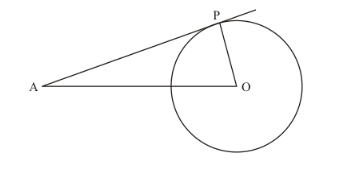# The length of the tangent from a point A at a circle, of radius 3 cm,

Question:

The length of the tangent from a point A at a circle, of radius 3 cm, is 4 cm. The distance of A from the centre of the circle is

(a) $\sqrt{7} \mathrm{~cm}$

(b) 7 cm

(c) 5 cm

(d) 25 cm

Solution:

Let us first put the given data in the form of a diagram.Given data is as follows:

OP = 3 cm

AP = 4 cm

We have to find the length of OA.

We know that the radius of a circle will always be perpendicular to the tangent at the point of contact. Therefore, OP is perpendicular to AP. We can now use Pythagoras theorem to find the length of OA.

$O A^{2}=O P^{2}+A P^{2}$

$O A^{2}=4^{2}+3^{2}$

$O A^{2}=16+9$

$O A^{2}=25$

$O A=5$

Therefore, the distance of the point A from the center of the circle is 5 cm.

Hence the correct answer is choice (c).